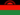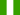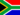Angolo somma dimostrazione

• Note di Apprendimento
• Revisione degli argomentiGregory C. 0 0 true that 3 angles of any triangle add up 180Ekeno U. 0 0 self practice on anlesRebecca C. 0 0 module: the sumbol for an angle is taken from a symbol of a tringle but it it must show only one angle, wow! that is interesting. topic: the sum of the angles in a triangle must be 180° wich is the. straight lineNkululeko M. 0 0 module: the sumbol for an angle is taken from a symbol of a tringle but it it must show only one angle, wow! that is interesting. topic: the sum of the angles in a triangle must be 180° wich is the. straight line
• Text Version